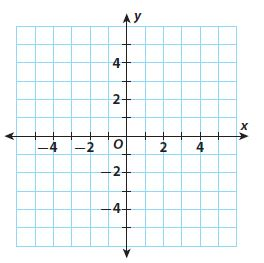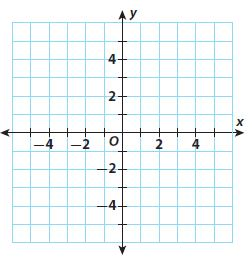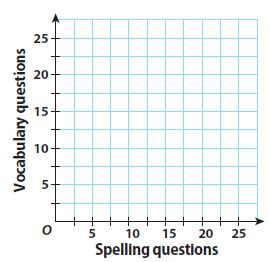Homework Explained - Math Practice 101Dear guest, you are not a registered member. As a guest, you only have read-only access to our books, tests and other practice materials.

As a registered member you can:

Registration is free and doesn't require any type of payment information. Click here to Register.
Go to page:
Chapter 8:Solving Systems of Linear Equations; Lesson1: Solving Systems of Linear Equations by Graphing

Guided Practice

Solve each system by graphing.

• Question 1

$$\begin{cases} y = 3x - 4\\ y = x + 2 \end{cases}$$• Type below:
• Question 2

$$\begin{cases} x - 3y = 2\\ -3x + 9y = -6 \end{cases}$$• Type below:
• Question 3

Mrs. Morales wrote a test with 15 questions covering spelling and vocabulary. Spelling questions (x) are worth 5 points and vocabulary questions (y) are worth 10 points. The maximum number of points possible on the test is 100.
a. Write an equation in slope-intercept form to represent the number of questions on the test.• Type below:
• Question 3

b. Write an equation in slope-intercept form to represent the total number of points on the test.

• Type below:
• Question 3

c. Graph the solutions of both equations.

• Type below:
• Question 3

d. Use your graph to tell how many of each question type are on the test.

•  spelling questions vocabulary questions

### ESSENTIAL QUESTION CHECK-IN

• Question 4

When you graph a system of linear equations, why does the intersection of the two lines represent the solution of the system?

• Type below:

Yes, email page to my online tutor. (if you didn't add a tutor yet, you can add one here)